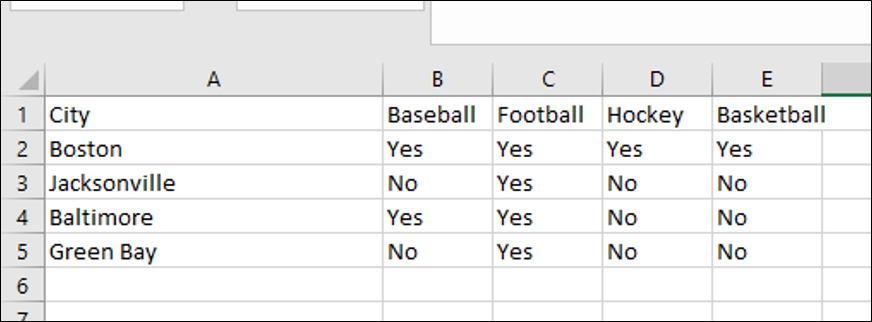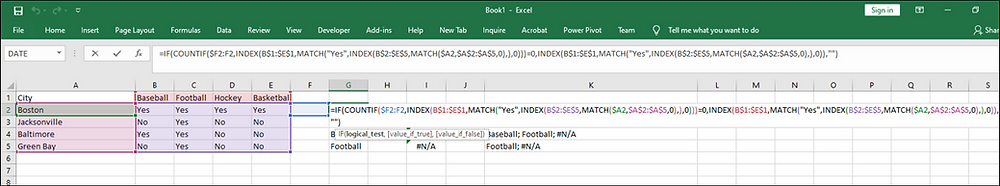Search

# Excel formula to pull column headings when given value is entered

Tonight's tip discusses how to use a formula in Excel to extract the column headings in a range of data for which a given value is entered. In this example we have a list of cities in column A, and columns B to E track which of these cities has a professional team for any one of the four major American sports.This formula is entered in cell G2:

=IF(COUNTIF(\$F2:F2,INDEX(B\$1:\$E\$1,MATCH("Yes",INDEX(B\$2:\$E\$5,MATCH(\$A2,\$A\$2:\$A\$5,0),),0)))=0,INDEX(B\$1:\$E\$1,MATCH("Yes",INDEX(B\$2:\$E\$5,MATCH(\$A2,\$A\$2:\$A\$5,0),),0)),"")The INDEX formula, with a nested MATCH formula, pulls an entry from the column headings in row 1, when the value 'Yes' is matched for the city in column A. The COUNTIF formula checks to see if the INDEX matches a value that the combined formula has found in the preceding cell. (The INDEX MATCH formula searches the full column range, not just one heading at a time, so there are no gaps in the list of heading hits.) The COUNTIF formula is written with the start of the range as an absolute cell reference, but the end of the range is not, so the range covered by the COUNTIF formula will expand as the formula is pulled to the right. The IF formula causes the result of the INDEX MATCH formula to be entered if the result is not already present.

Enter the formula two columns to the right of the data range to be reviewed. Pull it to the right using CTRL + R, for the same number of columns as exist in the data range, and then pull the formula down with CTRL + D. The values that get populated will only be those column headings that have "Yes" entered for a given city. You can then use the function discussed in the Tip of the Night for July 28, 2015, to combine the column headings and get a list of the sports in each city in a single cell like this: